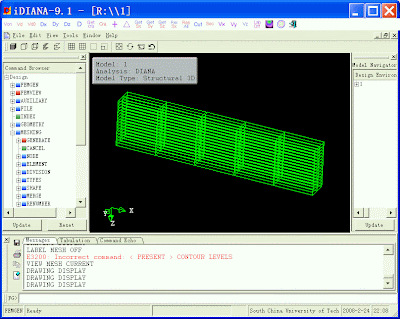## 2009年2月24日星期二

### 给DIANA软件添加图标工具栏

DIANA是个功能强大的非线性有限元分析软件，但是不得不说其前后处理确实还有很大的不足，虽然现在和MIDAS公司有了一定的合作，但是，大概还没有全盘改进吧。
1年前，在认真学习DIANA软件的时候，鉴于以上原因，给其加了一个丑陋的工具栏。不过也算改进了一下。


tip=Von Mises
image=Diana\von.png
leftaction=Send,RESULTS CALCULATE VONMISES{enter}
rightaction=
middleaction=[Settings]
class=QWidget
color=FFFFFF


tip=Von Mises
image=Diana\von.png
leftaction=Send,RESULTS CALCULATE VONMISES{enter}
rightaction=
middleaction=


tip=Deform
image=Diana\vd.png
leftaction=Send,VIEW OPTIONS DEFORM USING TDTX...G RESTDT 120{enter}
rightaction=
middleaction=


tip=Deform off
image=Diana\vd0.png
leftaction=Send,VIEW OPTIONS DEFORM USING TDTX...G RESTDT 0{enter}
rightaction=
middleaction=


tip=Displacement X
image=Diana\dx.png
leftaction=Send,RESULTS NODAL TDTX...G TDTX{enter}
rightaction=
middleaction=


tip=Displacement Y
image=Diana\dy.png
leftaction=Send,RESULTS NODAL TDTX...G TDTY{enter}
rightaction=
middleaction=


tip=Displacement Z
image=Diana\dz.png
leftaction=Send,RESULTS NODAL TDTX...G TDTZ{enter}
rightaction=
middleaction=


tip=Displacement All
image=Diana\d.png
leftaction=Send,RESULTS NODAL TDTX...G RESTDT{enter}
rightaction=
middleaction=


tip=Get crack
image=Diana\getcra.png
leftaction=Send,RESULTS GAUSSIAN EL.STCR1 STCRCK{enter}
rightaction=
middleaction=


tip=Present cra
image=Diana\cra.png
leftaction=Send,PRESENT SYMBOL{enter}
rightaction=
middleaction=


tip=+
image=Diana\+.png
leftaction=Send,PRESENT OPTIONS SYMBOL PLUS RANGE ALL{enter}
rightaction=
middleaction=


tip=triangle
image=Diana\sjx.png
leftaction=Send,PRESENT OPTIONS SYMBOL TRIANGLE RANGE ALL{enter}
rightaction=
middleaction=


tip=GET SX
image=Diana\getsx.png
leftaction=Send,RESULTS ELEMENT EL.SXX.G SXX{enter}
rightaction=
middleaction=


tip=GET SY
image=Diana\getsy.png
leftaction=Send,RESULTS ELEMENT EL.SXX.G SYY{enter}
rightaction=
middleaction=


tip=GET SZ
image=Diana\getsz.png
leftaction=Send,RESULTS ELEMENT EL.SXX.G SZZ{enter}
rightaction=
middleaction=


tip=GET rein SX
image=Diana\Reisx.png
leftaction=Send,RESULTS ELEMENT RE.SXX.G SXX{enter}
rightaction=
middleaction=


tip=All result
image=Diana\Resall.png
rightaction=
middleaction=


tip=Cut
image=Diana\cut.png
leftaction=Send,VIEW CUTAWAY CURSOR /CURSOR BOTTOM {enter}
rightaction=
middleaction=


tip=Section
image=Diana\sec.png
leftaction=Send,VIEW XSECTION CURSOR{enter}
rightaction=
middleaction=


tip=Vx
image=Diana\vx.png
leftaction=Send,EYE ANGLE 0 0 1{enter}
rightaction=
middleaction=


tip=Vy
image=Diana\vy.png
leftaction=Send,EYE ANGLE 0 90 0{enter}
rightaction=
middleaction=


tip=Vz
image=Diana\vz.png
leftaction=Send,EYE ANGLE 90 0 0{enter}
rightaction=
middleaction=


tip=label off
image=Diana\loff.png
leftaction=Send,LABEL MESH OFF{enter}
rightaction=
middleaction=


tip=Contour
image=Diana\contour.png
leftaction=Send,PRESENT CONTOUR LEVELS{enter}
rightaction=
middleaction=


tip=Contour line
image=Diana\conline.png
leftaction=Send,PRESENT OPTIONS CONTOUR LINES{enter}
rightaction=
middleaction=

### Geogebra的新功能http://support.microsoft.com/kb/290301

Microsoft 已更新了 Windows Installer 清理实用工具。利用 Windows Installer 清理实用工具，可以删除程序的 Windows Installer 配置信息。如果您遇到安装（安装程序）问题，可能需要删除程序的 Windows Installer 配置信息。例如，第一次安装程序时没有包括其中的某个组件，如果添加（或删除）此组件时遇到安装问题，您也许不得不删除该程序的 Windows Installer 配置信息。 Windows Installer 清理实用工具不执行下列功能：
• 删除 Windows Installer
• 删除由 Windows Installer 安装的任何程序（例如 Microsoft Office 2003）的文件
Windows Installer 清理实用工具可执行下列功能：
• 提供一个对话框，您可以从中选择一个或多个由 Windows Installer 安装的程序

为此，请在“Windows Installer 清理”对话框中的“已安装的产品”列表中选择所需程序。选择后，实用工具只删除与这些程序有关的 Windows Installer 配置信息。
• 删除构成所选程序的 Windows Installer 配置信息的文件和注册表设置我是选中了一个dwf ..的安装项删除的（找不到2007的相关项）

## 2009年1月16日星期五

### Autodesk公司的结构分析软件+Autodesk收购了ALGOR## 2008年11月7日星期五

### Web2.0 的连续梁计算程序

Web2.0 是不是就是应用程序网络化，不是特别懂啊

http://www.graitec.com/En/ePCCalc.asp## 2008年11月3日星期一

### 【TC技巧】如何将多个文件分别移入到以文件名命名的文件夹中

【TC技巧】如何将多个文件分别移入到以文件名命名的文件夹中
http://chenqj.blogspot.com

（1）将多个Pdf分别压缩到多个压缩文件中（按alt+F5，选中对每个文件分别创建压缩包，可选移动到压缩文件中）
（2）将多个压缩包解压（按alt+F9,选中将每个压缩文件解压到同名的文件夹中）

：），大功告成

## 2008年9月2日星期二

### 关于EXCEL里面的汉字转拼音首字母的函数

1. 采用定义名称和LOOKUP的方法

“={"","";"吖","A";"八","B";"嚓","C";"咑","D";"鵽","E";"发","F";"猤","G";"铪","H";" 夻","J";"咔","K";"垃","L";"嘸","M";"旀","N";"噢","O";"妑","P";"七","Q";"囕","R";" 仨","S";"他","T";"屲","W";"夕","X";"丫","Y";"帀","Z"}”

2. 自定义函数。
''''''''''''''''''''''
'VBscript代码提供者：小白
'联系方法：
'E-mail:xiaobai@17560.net
'
'VBA代码转换者：惟惟
'联系方法：Email:wuweiyin@yeah.net
'个人博客：http://weiwei.blog.163.com
'如有不正确的地方，请提出来或是帮忙完善
'谢谢
''''''''''''''''''''''''''''''
Function getpychar(char)
tmp = 65536 + Asc(char)
If (tmp >= 45217 And tmp <= 45252) Then
getpychar = "A"
ElseIf (tmp >= 45253 And tmp <= 45760) Then
getpychar = "B"
ElseIf (tmp >= 45761 And tmp <= 46317) Then
getpychar = "C"
ElseIf (tmp >= 46318 And tmp <= 46825) Then
getpychar = "D"
ElseIf (tmp >= 46826 And tmp <= 47009) Then
getpychar = "E"
ElseIf (tmp >= 47010 And tmp <= 47296) Then
getpychar = "F"
ElseIf (tmp >= 47297 And tmp <= 47613) Then
getpychar = "G"
ElseIf (tmp >= 47614 And tmp <= 48118) Then
getpychar = "H"
ElseIf (tmp >= 48119 And tmp <= 49061) Then
getpychar = "J"
ElseIf (tmp >= 49062 And tmp <= 49323) Then
getpychar = "K"
ElseIf (tmp >= 49324 And tmp <= 49895) Then
getpychar = "L"
ElseIf (tmp >= 49896 And tmp <= 50370) Then
getpychar = "M"
ElseIf (tmp >= 50371 And tmp <= 50613) Then
getpychar = "N"
ElseIf (tmp >= 50614 And tmp <= 50621) Then
getpychar = "O"
ElseIf (tmp >= 50622 And tmp <= 50905) Then
getpychar = "P"
ElseIf (tmp >= 50906 And tmp <= 51386) Then
getpychar = "Q"
ElseIf (tmp >= 51387 And tmp <= 51445) Then
getpychar = "R"
ElseIf (tmp >= 51446 And tmp <= 52217) Then
getpychar = "S"
ElseIf (tmp >= 52218 And tmp <= 52697) Then
getpychar = "T"
ElseIf (tmp >= 52698 And tmp <= 52979) Then
getpychar = "W"
ElseIf (tmp >= 52980 And tmp <= 53640) Then
getpychar = "X"
ElseIf (tmp >= 53689 And tmp <= 54480) Then
getpychar = "Y"
ElseIf (tmp >= 54481 And tmp <= 62289) Then
getpychar = "Z"
Else '如果不是中文，则不处理
getpychar = char
End If
End Function

Function getpy(str)
For i = 1 To Len(str)
getpy = getpy & getpychar(Mid(str, i, 1))
Next i
End Function

3.利用微软拼音（这个是整词转换的）

http://www.excelpx.com/home/show.aspx?id=199&cid=15

4.也有直接建立汉字和拼音的对应库表，直接查询的。：）

## 2008年8月31日星期日

1） Slowmousion: slow down the mouse via hotkey for a higher degree of control

http://www.freewaregenius.com/2008/08/27/slowmousion-slow-down-the-mouse-via-hotkey-for-a-higher-degree-of-control/2）CRP: automatically re-launch apps and control a program’s allowed number of running instances

http://www.freewaregenius.com/2008/07/14/crp-automatically-re-launch-apps-and-control-a-programs-allowed-number-of-running-instances/3）Phlox: use “f2″ in XP to rename files without overwriting file extensions

http://www.freewaregenius.com/2008/08/15/phlox-use-f2-in-xp-to-rename-files-without-overwriting-file-extensions/4）“Types”: easily configure the default apps, icons, and context menu entries for file types